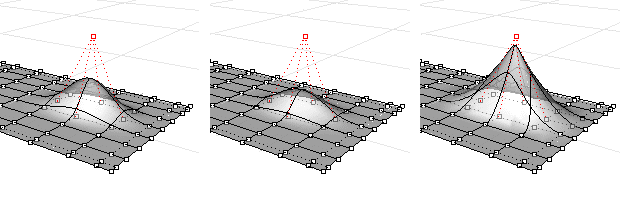# Weight

The Weight command edits the weight of a curve or surface control point.

The weight of a control point determines how much the curve or surface is attracted to the control point. The higher the value of the weight, the closer the curve or surface is to the control point.

Warning: If you are exporting to another application, it is best to leave all control point weights at 1.

Steps

Use the slider to adjust the weight of the selected control points.

Set Control Point Weight

Slider and Edit box

Set weight between 0.1 and 10.0.

ExampleWeight=1 (left), Weight=0.5 (center), Weight=10 (right).